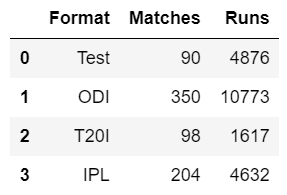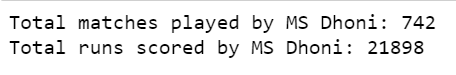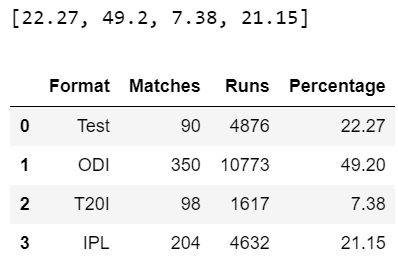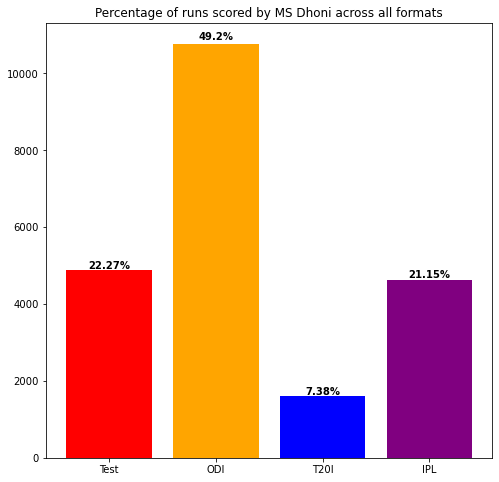GeeksforGeeks App
Open AppBrowser
Continue

# Display percentage above bar chart in Matplotlib

In this article, we are going to discuss how we can plot a bar chart using the Matplotlib library and display percentages above each bar in the bar chart.

For the sake of explanation, we are going to take an example of the runs scored by former Indian Captain MS Dhoni across all the formats, and then we will compare the percentage of runs scored across all formats with respect to the total runs scored by him. Let us start with importing the necessary libraries at first and then making a dataframe containing the runs scored in each format.

## Python

 `# import modules``import` `pandas as pd``import` `matplotlib.pyplot as plt` `# assign data``data ``=` `pd.DataFrame({``'Format'``: [``'Test'``, ``'ODI'``, ``'T20I'``, ``'IPL'``],``                     ``'Matches'``: [``90``, ``350``, ``98``, ``204``],``                     ``'Runs'``: [``4876``, ``10773``, ``1617``, ``4632``]``                     ``})` `# display data``display(data)`

Output:Now, let us calculate the total matches played by him and the total runs scored by him across all the formats.

## Python

 `# get total matches of``# a particular player``total_matches ``=` `data.Matches.``sum``()``print``(``'Total matches played by MS Dhoni: %s'` `%` `total_matches)` `# get total runs of``# a particular player``total_runs ``=` `data.Runs.``sum``()``print``(``'Total runs scored by MS Dhoni: %s'` `%` `total_runs)`

Output:Calculating the percentage of runs scored across all formats with respect to total runs and add the percentage field in our original dataframe which can be done as:

## Python

 `# compute percentage of each format``percentage ``=` `[]` `for` `i ``in` `range``(data.shape[``0``]):``    ``pct ``=` `(data.Runs[i] ``/` `total_runs) ``*` `100``    ``percentage.append(``round``(pct, ``2``))` `# display percentage``print``(percentage)` `# display data``data[``'Percentage'``] ``=` `percentage``display(data)`

Output:Now, that we have all our data ready, we can start with plotting our bar plot and later displaying the respective percentage of runs scored across each format over each bar in the bar chart.

We can use the plt.bar() method present inside the matplotlib library to plot our bar graph. We are passing here three parameters inside the plt.bar() method that corresponds to X-axis values (Format), Y-axis values (Runs) and the colors that we want to assign to each bar in the bar plot. The plt.bar() method also returns the coordinates of the rectangles in the bar chart. We can make an object named ‘graph‘ that will be used to store these coordinates and later we can retrieve the coordinates using a for loop iterating on this object. We can use get_width() method and get_height() method that returns the width and height of each bar respectively in the bar graph. Also, get_xy() method can be used to get the x and y coordinates at the bottom leftmost point of each bar.

Later, we can use the plt.text() method to put the desired text (here percentage) on the plot, and we can pass the x and y position and the string we want to write inside the plt.text() method. It will write the desired percentage on the bar chart.

## Python

 `# depict illustration``plt.figure(figsize``=``(``8``, ``8``))``colors_list ``=` `[``'Red'``, ``'Orange'``, ``'Blue'``, ``'Purple'``]``graph ``=` `plt.bar(data.``Format``, data.Runs, color``=``colors_list)``plt.title(``'Percentage of runs scored by MS Dhoni across all formats'``)` `i ``=` `0``for` `p ``in` `graph:``    ``width ``=` `p.get_width()``    ``height ``=` `p.get_height()``    ``x, y ``=` `p.get_xy()``    ` `    ``plt.text(x``+``width``/``2``,``             ``y``+``height``*``1.01``,``             ``str``(data.Percentage[i])``+``'%'``,``             ``ha``=``'center'``,``             ``weight``=``'bold'``)``    ``i ``+``=` `1``plt.show()`

When we call the plt.show() method our bar will be represented.

Below is the complete program based on the above approach:

## Python

 `# import modules``import` `pandas as pd``import` `matplotlib.pyplot as plt` `# assign data``data ``=` `pd.DataFrame({``'Format'``:[``'Test'``,``'ODI'``,``'T20I'``,``'IPL'``],``                     ``'Matches'``: [``90``, ``350``, ``98``, ``204``],``                     ``'Runs'``:[``4876``,``10773``, ``1617``, ``4632``]``                    ``})`  `# compute percentage of each format``percentage ``=` `[]``for` `i ``in` `range``(data.shape[``0``]):``    ``pct ``=` `(data.Runs[i] ``/` `total_runs) ``*` `100``    ``percentage.append(``round``(pct,``2``))``data[``'Percentage'``] ``=` `percentage` `# depict illustration``plt.figure(figsize``=``(``8``,``8``))``colors_list ``=` `[``'Red'``,``'Orange'``, ``'Blue'``, ``'Purple'``]``graph ``=` `plt.bar(data.``Format``,data.Runs, color ``=` `colors_list)``plt.title(``'Percentage of runs scored by MS Dhoni across all formats'``)` `i ``=` `0``for` `p ``in` `graph:``    ``width ``=` `p.get_width()``    ``height ``=` `p.get_height()``    ``x, y ``=` `p.get_xy()``    ``plt.text(x``+``width``/``2``,``             ``y``+``height``*``1.01``,``             ``str``(data.Percentage[i])``+``'%'``,``             ``ha``=``'center'``,``             ``weight``=``'bold'``)``    ``i``+``=``1``plt.show()`

Output:My Personal Notes arrow_drop_up